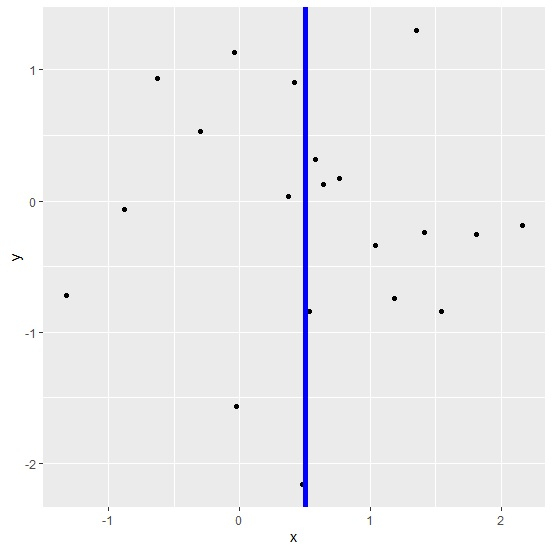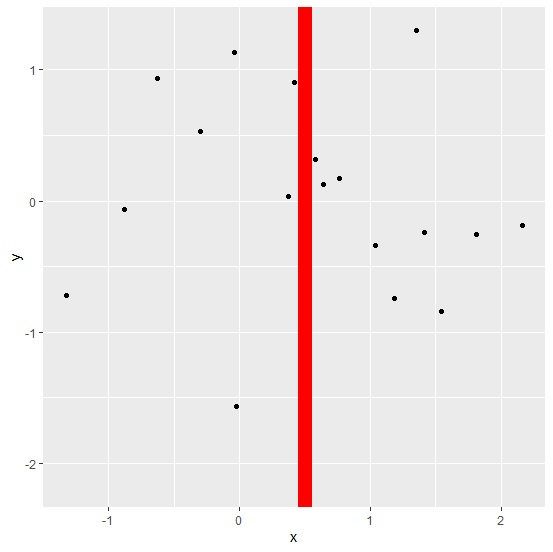# How to create a wide vertical line using ggplot2 with different color in R?

To create a vertical line using ggplot2, we can use geom_vline function of ggplot2 package and if we want to have a wide vertical line with different color then lwd and colour argument will be used. The lwd argument will increase the width of the line and obviously colour argument will change the color.

Check out the below given example to understand how it works.

## Example

Following snippet creates a sample data frame −

x<-rnorm(20)
y<-rnorm(20)
df<-data.frame(x,y)
df

The following dataframe is created −

       x            y
1   1.35002649   1.30387113
2   1.03471773  -0.34014609
3   1.18443924  -0.74579071
4  -0.88285300  -0.06256158
5   0.64281609   0.12690947
6  -0.29592691   0.52991361
7  -0.02380484  -1.56435084
8   0.42122270   0.90217653
9   1.80724368  -0.25452147
10  0.37268981   0.03689309
11  1.54166527  -0.84113791
12 -0.62766477   0.93255809
13 -1.32431288  -0.72187512
14 -0.03774817   1.13166647
15  1.41402735  -0.24267547
16  2.16421759  -0.18939033
17  0.53090455  -0.84479543
18  0.57897106   0.32036809
19  0.76414767   0.16915787
20  0.48237580  -2.16234466

To load ggplot2 package and create point chart between x and y with wide vertical line at X=0.5 in blue color, add the following code to the above snippet −

library(ggplot2)
ggplot(df,aes(x,y))+geom_point()+geom_vline(xintercept=0.5,lwd=2,colour="blue")

## Output

If you execute all the above given snippets as a single program, it generates the following output: −Now, to create point chart between x and y with wide vertical line at X=0.5 in red color, add the following code to the above snippet −

ggplot(df,aes(x,y))+geom_point()+geom_vline(xintercept=0.5,lwd=5,colour="red")


## Output

If you execute all the above given snippets as a single program, it generates the following output: −